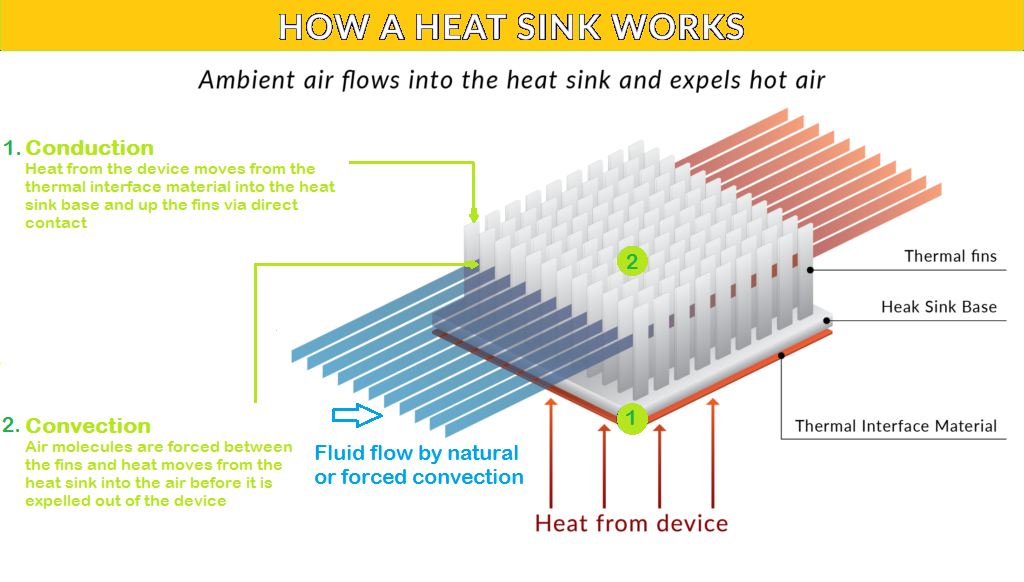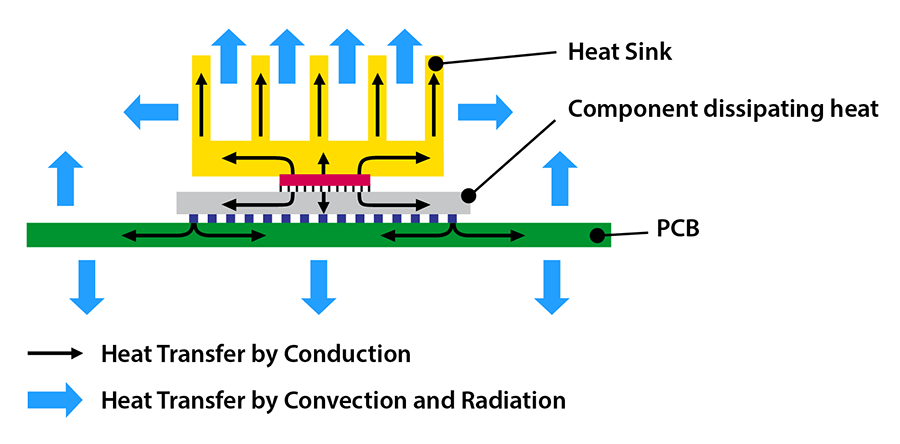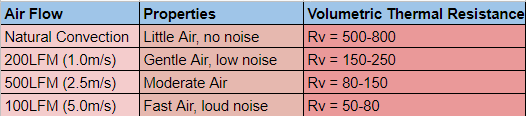# HEAT SINK

A heat sink is a passive heat exchanger that transfers the heat generated by an electronic or a mechanical device to fluid medium, often air or a liquid coolant, where it is dissipated away from the device, thereby allowing regulation of the device's temperature. In computers, heat sinks are used to cool CPUs, GPUs, and some chipsets and RAM modules. Heat sinks are used with high-power semiconductor devices such as power transistors and optoelectronics such as lasers and light-emitting diodes (LEDs), where the heat dissipation ability of the component itself is insufficient to moderate its temperature.

For any electronics device, “Thermal Management” is the important part which decides the performance and efficiency of the device during its entire product life. This thing will highlight into the Selection and Design of Heat Sinks.

How heat is Transferred ?

The Heat is transferred from higher temperature to lower temperature by the following 3 Ways-

Conduction: It happens due to movement of molecules. Molecules at higher temperatures vibrate at a higher amplitude and pass the energy to the lower temperature molecules.

Convection: Heat is transferred from a hot surface to a cool surface by the bulk movement of fluids (air or liquid).

Most of the electronic device cooling systems use conduction and convection type heat transfer for thermal management.

Determine Power Dissipation by the Device:

The first step in determining the Heat Sink design is to figure out how much power the Semiconductor device dissipates. There are different methodologies applied to calculate the Power Loss in the Semiconductor device. The below formula provides information about how the device power dissipation can be calculated.

Power Dissipation = (Vin – Vout) * Iout

eg, If the 7805 input is 9V and it is providing 2A of current, then Power Dissipation = (9V – 5V) * 2A = 8W.

Extract Datasheet Values:

The next step after obtaining the device power dissipation is extracting thermal resistance values from the Datasheet of the component.

As per the datasheet of Posstive-Voltage regulators, the maximum operating virtual junction temperature Tj = 125˚C. So under any operating conditions, the Tj should be managed below 125˚C.

After determining the maximum junction temperature rating, the device's thermal resistance must be determined. To evaluate the thermal resistance, we must first finalize the sort of packaging that will be used for our design. The junction-to-ambient thermal resistance R(JA) = 19 °C/W TO-220 package is chosen.

Calculating the maximum junction temperature without a heat sink-

Tj = Ta + Rө(JA) * Power

Ta = Ambient Temperature (Environment Temperature)

Tj = 35 + (19 * 8)  (assuming Ta = 35)

= 187˚C

As per the calculation, if the device is used without a heat sink, the maximum junction temperature of the device reaching 187˚C. This should be reduced below 125˚C and hence the Heat Sink should be used.

Obtaining Junction to Case Thermal Resistance Value:

The below image provides details about the different sections of the semiconductor thermal system. The Rө(JC) can be obtained from the device datasheet (here LM7805). For the LM7805 - TO-220 package, the junction to case temperature is 3˚C/W.Choosing a Correct Heat Sink for your Component by Calculating the size of heat sink.

Heat Source Power (Q): This is the thermal design power (TDP) of the chip, which is the maximum amount of heat it can create in watts without going outside its thermal envelope. It should be given by the chip manufacturer or an ASIC engineer.

Tc Max: The chip case's maximum temperature. The manufacturer will usually give this for most chip designs. The maximum Tj (junction temperature) for bare die chips will be provided. Use the Tj spec instead of Tc max in this case.

Max Ambient: The maximum ambient temperature at which the device is intended to operate.

Thermal Budget: (Tc Max - Max Ambient). The sum total of all ΔTs in the network, from Tc to Air temperature rise cannot exceed this limit.

Note – thermal budgets below 40 degrees Celsius are generally good candidates for two-phase cooling using heat pipes or vapor chambers.

Volumetric Thermal Resistance (Rv): This equation and subsequent guidelines have been shown to closely estimate heat sink volume:

V=(Q*Rv)/ΔT.

The first step in using the chart below is to know the available airflow across the heat sink. As you know, the higher the airflow the smaller the heat sink. The first challenge you’ll probably face is that the fan manufacturer has given you the ‘bulk airflow’, usually in cubic feet per minute (CFM), but not the air velocity. It’s easy to determine the velocity if you know the size of the heat sink.

Here are some rough guidelines:

• Open air natural convection develops about 40 LFM which is about just enough to blow out a match.
• 1 m/s or 200 LFM you can feel the flow but not hear it.
• 2.5 m/s or 500 LFM is a good flow that will blow out a whole bunch of candles and you can begin to hear the noise, especially in a quiet environment.
• 5 m/s or 1000 LFM is going to be noisy. Not to be used in any noise-sensitive environment.Firstly you have to select the appropriate airflow, the next step is choosing from a range of Rv. In the case of moderate air (2.5 m/s) the range is 80-150. The published rule is as follows:

• For heat sinks smaller than 300 cm³ you use the lower limit, in this case 80.
• For large heat sinks, greater than 1,000 cm³ use the upper limit.

If you initially use 110 Rv value and the estimated volume of the heat sink is less than 300 cm³, change the Rv to 80, which is the lower end of the Rv value for moderate airflow.

When designing devices that must work at altitude, it’s important to de-rate the Rv. A solid rule of thumb is 10% for every mile of altitude. For example, at one mile high we’d divide 80 Rv by 0.9 to end up with a de-rated Rv of roughly 89.

After volume of heat sink is determined, the last step is to assign some length, width and height dimensions. It is generally depend on the space available to attach the heat sink.

There are two types of heat sinks: active and passive. Example Peltier Heat Sink TEC1-12706 is a Suitable for a Thermoelectric cooling system.

Factors to Improve Thermal Performance of a Heat Sink

1. Heat Sink Design: Shape and Size of Fins and there Arrangement.
2. Airflow Direction: Airflow should be parallel to the fins
3. Air Speed and Temperature
4. Heat Sink Material: Copper or Aluminium
5. Thermal Interface Material: Heat Conductive Paste
6. Thermal Resistance of Heat Sink
Learn